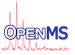OpenMS
RansacModelLinear Class Reference

Implementation of a linear RANSAC model fit. More...

#include <OpenMS/MATH/MISC/RANSACModelLinear.h>

Inheritance diagram for RansacModelLinear:
[legend]
Collaboration diagram for RansacModelLinear:
[legend]

## Static Public Member Functions

static ModelParameters rm_fit_impl (const DVecIt &begin, const DVecIt &end)

static double rm_rsq_impl (const DVecIt &begin, const DVecIt &end)

static double rm_rss_impl (const DVecIt &begin, const DVecIt &end, const ModelParameters &coefficients)

static DVec rm_inliers_impl (const DVecIt &begin, const DVecIt &end, const ModelParameters &coefficients, double max_threshold)Public Types inherited from RansacModel< RansacModelLinear >
typedef std::pair< double, double > DPair

typedef std::vector< DPairDVec

typedef DVec::const_iterator DVecIt

typedef std::vector< double > ModelParametersPublic Member Functions inherited from RansacModel< RansacModelLinear >
ModelParameters rm_fit (const DVecIt &begin, const DVecIt &end) const
fit a model and return its parameters More...

double rm_rsq (const DVecIt &begin, const DVecIt &end) const
Returns the R-squared of the data applied to the model (computed on-the-fly). More...

double rm_rss (const DVecIt &begin, const DVecIt &end, const ModelParameters &coefficients) const
calculates the residual sum of squares of the input points according to the model More...

DVec rm_inliers (const DVecIt &begin, const DVecIt &end, const ModelParameters &coefficients, double max_threshold) const

## Detailed Description

Implementation of a linear RANSAC model fit.

Using generic plug-in template base class 'RansacModel' using 'Curiously recurring template pattern' (CRTP).

## ◆ rm_fit_impl()

 static ModelParameters rm_fit_impl ( const DVecIt & begin, const DVecIt & end )
static

## ◆ rm_inliers_impl()

 static DVec rm_inliers_impl ( const DVecIt & begin, const DVecIt & end, const ModelParameters & coefficients, double max_threshold )
static

## ◆ rm_rsq_impl()

 static double rm_rsq_impl ( const DVecIt & begin, const DVecIt & end )
static